Levels »

# Charon Doesn’t Make Change

### Demo 2.0, Elevation: 3.0m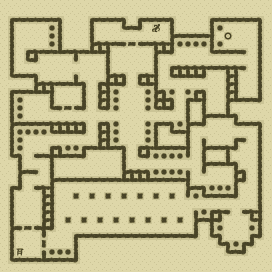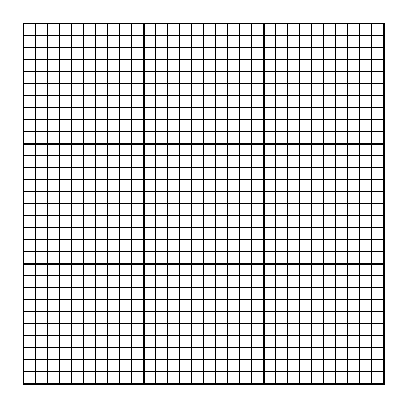### Items

•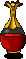Bubbling Red Potion (4, 2)
•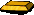Gold Ingot (4, 3)
•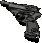Walther P4 Pistol (18, 2) (on Anonymous German)
•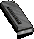18 Walther P4 Magazines (144 rounds) (18, 2) x8 (in Walther P4 Pistol) (on Anonymous German) - (18, 2) x8 (on Anonymous German) - (18, 2) x8 (on Anonymous German) - (18, 2) x8 (on Anonymous German) - (18, 2) x8 (on Anonymous German) - (30, 2) x8 - (30, 2) x8 - (4, 3) x8 - (4, 3) x8 - (25, 8) x8 - (29, 8) x8 - (2, 11) x8 - (14, 12) x8 - (16, 15) x8 - (8, 22) x8 - (8, 22) x8 - (18, 27) x8 - (27, 28) x8
•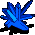Blue Crystal (26, 3) (60 uses left)

•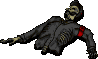(18, 2) Anonymous German
•Walther P4 Pistol
•Walther P4 Magazine x8
•Walther P4 Magazine x8
•Walther P4 Magazine x8
•Walther P4 Magazine x8
•Walther P4 Magazine x8

### Monsters

•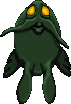4 Nightmares (27, 2) - (27, 4) - (6, 22) - (6, 24)
•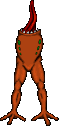28 Headless (Random rate: 8) (2, 3) - (15, 3) - (17, 3) - (21, 5) - (22, 5) - (22, 5) - (30, 6) - (26, 9) - (6, 10) - (8, 10) - (25, 10) - (2, 12) - (3, 12) - (28, 18) - (2, 19) - (27, 20) - (26, 21) - (2, 22) - (11, 23) - (17, 23) - (10, 26) - (16, 26) - (20, 26) - (27, 27) - (29, 27) - (28, 28) - (6, 29) - (7, 29)
•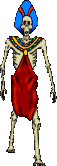6 Zombies (Random rate: 4) (3, 2) - (27, 3) - (3, 4) - (23, 5) - (13, 25) - (6, 28)

### Locations

• Door closer (2, 26)
• 4 Door openers (15, 5) - (7, 13) - (2, 28) - (5, 29)
• 32 Do-not-disturb sectors(2, 2) - (3, 2) - (4, 2) - (27, 2) - (28, 2) - (2, 3) - (3, 3) - (4, 3) - (28, 3) - (2, 4) - (3, 4) - (4, 4) - (27, 4) - (28, 4) - (21, 5) - (22, 5) - (23, 5) - (24, 5) - (30, 6) - (28, 18) - (2, 19) - (26, 20) - (27, 20) - (26, 21) - (27, 21) - (27, 27) - (28, 27) - (29, 27) - (6, 28) - (28, 28) - (6, 29) - (7, 29)
• Save rune(27, 3)